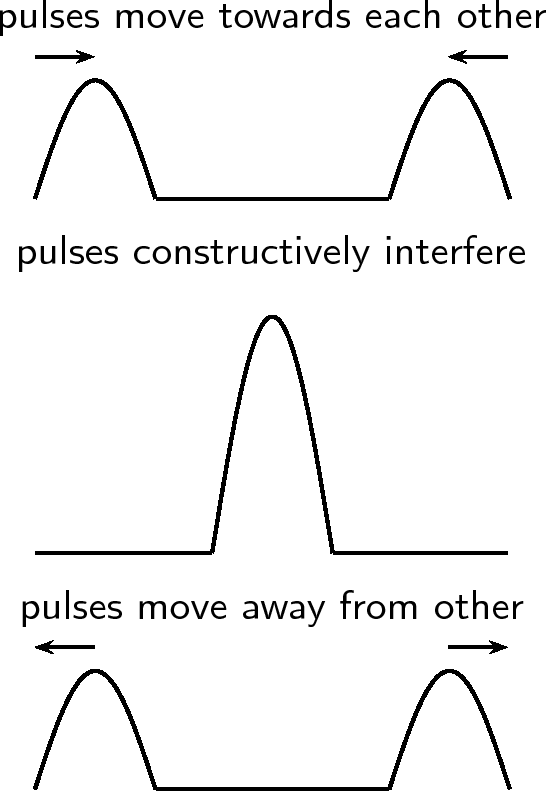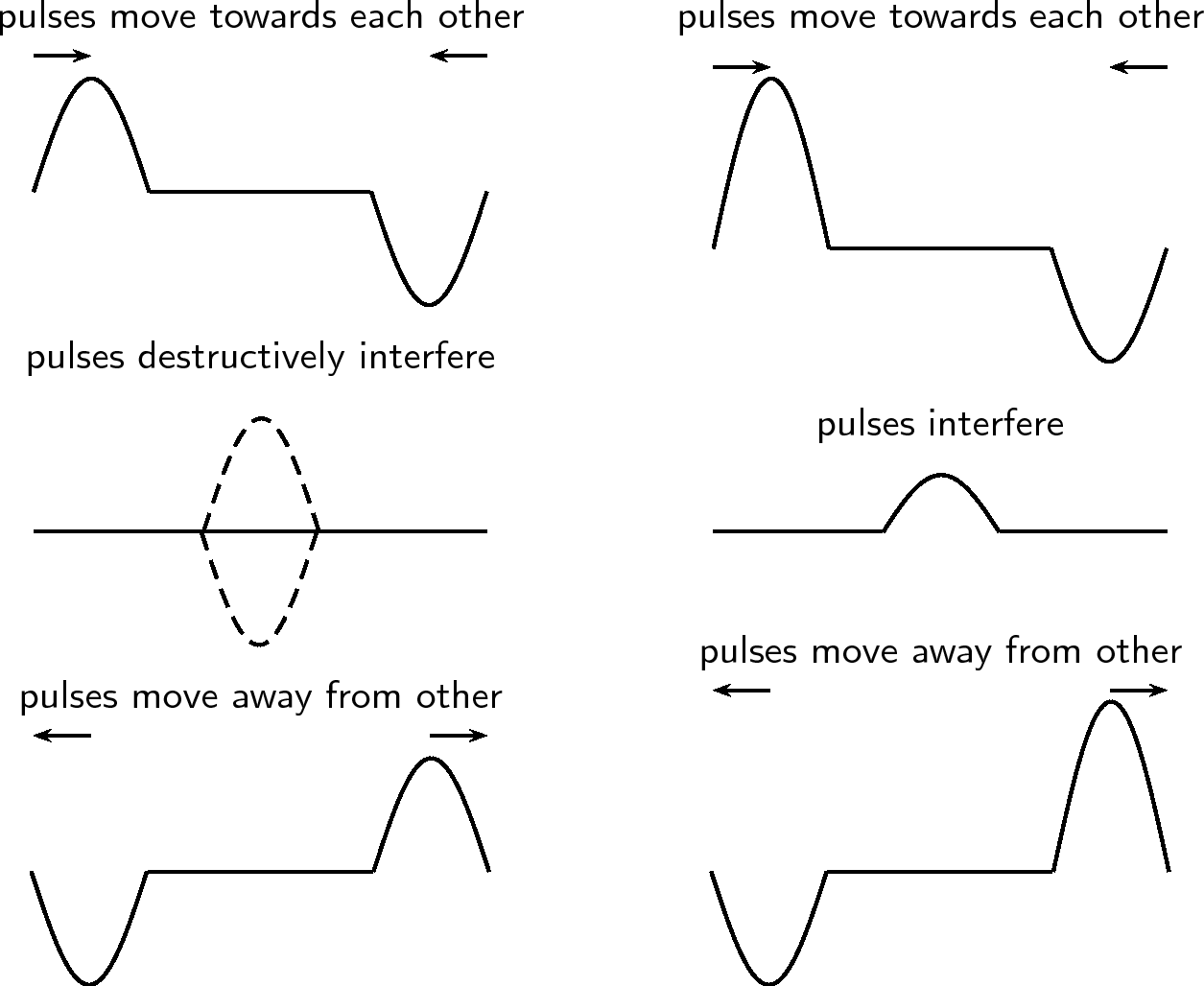# 6.1 Superposition of pulses

 Page 1 / 1

## Superposition of pulses

Two or more pulses can pass through the same medium at that same time in the same place. When they do they interact with each other to form a different disturbance at that point. The resulting pulse is obtained by using the principle of superposition . The principle of superposition states that the effect of the different pulses is the sum of their individual effects. After pulses pass through each other, each pulse continues along its original direction of travel, and their original amplitudes remain unchanged.

Constructive interference takes place when two pulses meet each other to create a larger pulse. The amplitude of the resulting pulse is the sum of the amplitudes of the two initial pulses. This is shown in [link] .

Constructive interference
Constructive interference is when two pulses meet, resulting in a bigger pulse.Superposition of two pulses: constructive interference.

Destructive interference takes place when two pulses meet and cancel each other. The amplitude of the resulting pulse is the sum of the amplitudes of the two initial pulses, but the one amplitude will be a negative number. This is shown in [link] . In general, amplitudes of individual pulses add together to give the amplitude of the resultant pulse.

Destructive interference
Destructive interference is when two pulses meet, resulting in a smaller pulse.Superposition of two pulses. The left-hand series of images demonstrates destructive interference, since the pulses cancel each other. The right-hand series of images demonstrate a partial cancelation of two pulses, as their amplitudes are not the same in magnitude.

The two pulses shown below approach each other at $1\phantom{\rule{2pt}{0ex}}\mathrm{m}·\mathrm{s}{}^{-1}$ . Draw what the waveform would look like after $1\phantom{\rule{2pt}{0ex}}\mathrm{s}$ , $2\phantom{\rule{2pt}{0ex}}\mathrm{s}$ and $5\phantom{\rule{2pt}{0ex}}\mathrm{s}$ .

1. After $1\phantom{\rule{2pt}{0ex}}\mathrm{s}$ , pulse A has moved $1\phantom{\rule{2pt}{0ex}}\mathrm{m}$ to the right and pulse B has moved $1\phantom{\rule{2pt}{0ex}}\mathrm{m}$ to the left.

2. After $1\phantom{\rule{2pt}{0ex}}\mathrm{s}$ more, pulse A has moved $1\phantom{\rule{2pt}{0ex}}\mathrm{m}$ to the right and pulse B has moved $1\phantom{\rule{2pt}{0ex}}\mathrm{m}$ to the left.

3. After $5\phantom{\rule{2pt}{0ex}}\mathrm{s}$ , pulse A has moved $5\phantom{\rule{2pt}{0ex}}\mathrm{m}$ to the right and pulse B has moved $5\phantom{\rule{2pt}{0ex}}\mathrm{m}$ to the left.

The idea of superposition is one that occurs often in physics. You will see much, much more of superposition!

## Aim

To demonstrate constructive and destructive interference

## Apparatus

Ripple tank apparatus

## Method

1. Set up the ripple tank
2. Produce a single pulse and observe what happens
3. Produce two pulses simultaneously and observe what happens
4. Produce two pulses at slightly different times and observe what happens

## Results and conclusion

You should observe that when you produce two pulses simultaneously you see them interfere constructively and when you produce two pulses at slightly different times you see them interfere destructively.

## Problems involving superposition of pulses

1. For the following pulse, draw the resulting wave forms after $1\phantom{\rule{2pt}{0ex}}\mathrm{s}$ , $2\phantom{\rule{2pt}{0ex}}\mathrm{s}$ , $3\phantom{\rule{2pt}{0ex}}\mathrm{s}$ , $4\phantom{\rule{2pt}{0ex}}\mathrm{s}$ and $5\phantom{\rule{2pt}{0ex}}\mathrm{s}$ . Each pulse is travelling at $1\phantom{\rule{2pt}{0ex}}\mathrm{m}·\mathrm{s}{}^{-1}$ . Each block represents $1\phantom{\rule{2pt}{0ex}}\mathrm{m}$ . The pulses are shown as thick black lines and the undisplaced medium as dashed lines.
2. For the following pulse, draw the resulting wave forms after $1\phantom{\rule{2pt}{0ex}}\mathrm{s}$ , $2\phantom{\rule{2pt}{0ex}}\mathrm{s}$ , $3\phantom{\rule{2pt}{0ex}}\mathrm{s}$ , $4\phantom{\rule{2pt}{0ex}}\mathrm{s}$ and $5\phantom{\rule{2pt}{0ex}}\mathrm{s}$ . Each pulse is travelling at $1\phantom{\rule{2pt}{0ex}}\mathrm{m}·\mathrm{s}{}^{-1}$ . Each block represents $1\phantom{\rule{2pt}{0ex}}\mathrm{m}$ . The pulses are shown as thick black lines and the undisplaced medium as dashed lines.
3. For the following pulse, draw the resulting wave forms after $1\phantom{\rule{2pt}{0ex}}\mathrm{s}$ , $2\phantom{\rule{2pt}{0ex}}\mathrm{s}$ , $3\phantom{\rule{2pt}{0ex}}\mathrm{s}$ , $4\phantom{\rule{2pt}{0ex}}\mathrm{s}$ and $5\phantom{\rule{2pt}{0ex}}\mathrm{s}$ . Each pulse is travelling at $1\phantom{\rule{2pt}{0ex}}\mathrm{m}·\mathrm{s}{}^{-1}$ . Each block represents $1\phantom{\rule{2pt}{0ex}}\mathrm{m}$ . The pulses are shown as thick black lines and the undisplaced medium as dashed lines.
4. For the following pulse, draw the resulting wave forms after $1\phantom{\rule{2pt}{0ex}}\mathrm{s}$ , $2\phantom{\rule{2pt}{0ex}}\mathrm{s}$ , $3\phantom{\rule{2pt}{0ex}}\mathrm{s}$ , $4\phantom{\rule{2pt}{0ex}}\mathrm{s}$ and $5\phantom{\rule{2pt}{0ex}}\mathrm{s}$ . Each pulse is travelling at $1\phantom{\rule{2pt}{0ex}}\mathrm{m}·\mathrm{s}{}^{-1}$ . Each block represents $1\phantom{\rule{2pt}{0ex}}\mathrm{m}$ . The pulses are shown as thick black lines and the undisplaced medium as dashed lines.
5. For the following pulse, draw the resulting wave forms after $1\phantom{\rule{2pt}{0ex}}\mathrm{s}$ , $2\phantom{\rule{2pt}{0ex}}\mathrm{s}$ , $3\phantom{\rule{2pt}{0ex}}\mathrm{s}$ , $4\phantom{\rule{2pt}{0ex}}\mathrm{s}$ and $5\phantom{\rule{2pt}{0ex}}\mathrm{s}$ . Each pulse is travelling at $1\phantom{\rule{2pt}{0ex}}\mathrm{m}·\mathrm{s}{}^{-1}$ . Each block represents $1\phantom{\rule{2pt}{0ex}}\mathrm{m}$ . The pulses are shown as thick black lines and the undisplaced medium as dashed lines.
6. For the following pulse, draw the resulting wave forms after $1\phantom{\rule{2pt}{0ex}}\mathrm{s}$ , $2\phantom{\rule{2pt}{0ex}}\mathrm{s}$ , $3\phantom{\rule{2pt}{0ex}}\mathrm{s}$ , $4\phantom{\rule{2pt}{0ex}}\mathrm{s}$ and $5\phantom{\rule{2pt}{0ex}}\mathrm{s}$ . Each pulse is travelling at $1\phantom{\rule{2pt}{0ex}}\mathrm{m}·\mathrm{s}{}^{-1}$ . Each block represents $1\phantom{\rule{2pt}{0ex}}\mathrm{m}$ . The pulses are shown as thick black lines and the undisplaced medium as dashed lines.
7. What is superposition of waves?
8. What is constructive interference?
9. What is destructive interference?

The following presentation provides a summary of the work covered in this chapter. Although the presentation is titled waves, the presentation covers pulses only.

## Summary

• A medium is the substance or material in which a wave will move
• A pulse is a single disturbance that moves through a medium
• The amplitude of a pules is a measurement of how far the medium is displaced from rest
• Pulse speed is the distance a pulse travels per unit time
• Constructive interference is when two pulses meet and result in a bigger pulse
• Destructive interference is when two pulses meet and and result in a smaller pulse
• We can draw graphs to show the motion of a particle in the medium or to show the motion of a pulse through the medium
• When a pulse moves from a thin rope to a thick rope, the speed and pulse length decrease. The pulse will be reflected and inverted in the thin rope. The reflected pulse has the same length and speed, but a different amplitude
• When a pulse moves from a thick rope to a thin rope, the speed and pulse length increase. The pulse will be reflected in the thick rope. The reflected pulse has the same length and speed, but a different amplitude
• A pulse reaching a free end will be reflected but not inverted. A pulse reaching a fixed end will be reflected and inverted

## Exercises - transverse pulses

1. A heavy rope is flicked upwards, creating a single pulse in the rope. Make a drawing of the rope and indicate the following in your drawing:
1. The direction of motion of the pulse
2. Amplitude
3. Pulse length
4. Position of rest
2. A pulse has a speed of $2,5\phantom{\rule{2pt}{0ex}}\mathrm{m}·\mathrm{s}{}^{-1}$ . How far will it have travelled in $6\phantom{\rule{2pt}{0ex}}\mathrm{s}$ ?
3. A pulse covers a distance of $75\phantom{\rule{2pt}{0ex}}\mathrm{cm}$ in $2,5\phantom{\rule{2pt}{0ex}}\mathrm{s}$ . What is the speed of the pulse?
4. How long does it take a pulse to cover a distance of $200\phantom{\rule{2pt}{0ex}}\mathrm{mm}$ if its speed is $4\phantom{\rule{2pt}{0ex}}\mathrm{m}·\mathrm{s}{}^{-1}$ ?
5. The following position-time graph for a pulse in a slinky spring is given. Draw an accurate sketch graph of the velocity of the pulse against time.
6. The following velocity-time graph for a particle in a medium is given. Draw an accurate sketch graph of the position of the particle vs. time.
7. Describe what happens to a pulse in a slinky spring when:
1. the slinky spring is tied to a wall.
2. the slinky spring is loose, i.e. not tied to a wall.
8. The following diagrams each show two approaching pulses. Redraw the diagrams to show what type of interference takes place, and label the type of interference.
9. Two pulses, A and B, of identical shape and amplitude are simultaneously generated in two identical wires of equal mass and length. Wire A is, however, pulled tighter than wire B. Which pulse will arrive at the other end first, or will they both arrive at the same time?

#### Questions & Answers

Is there any normative that regulates the use of silver nanoparticles?
what king of growth are you checking .?
Renato
What fields keep nano created devices from performing or assimulating ? Magnetic fields ? Are do they assimilate ?
why we need to study biomolecules, molecular biology in nanotechnology?
?
Kyle
yes I'm doing my masters in nanotechnology, we are being studying all these domains as well..
why?
what school?
Kyle
biomolecules are e building blocks of every organics and inorganic materials.
Joe
anyone know any internet site where one can find nanotechnology papers?
research.net
kanaga
sciencedirect big data base
Ernesto
Introduction about quantum dots in nanotechnology
what does nano mean?
nano basically means 10^(-9). nanometer is a unit to measure length.
Bharti
do you think it's worthwhile in the long term to study the effects and possibilities of nanotechnology on viral treatment?
absolutely yes
Daniel
how to know photocatalytic properties of tio2 nanoparticles...what to do now
it is a goid question and i want to know the answer as well
Maciej
characteristics of micro business
Abigail
for teaching engĺish at school how nano technology help us
Anassong
Do somebody tell me a best nano engineering book for beginners?
there is no specific books for beginners but there is book called principle of nanotechnology
NANO
what is fullerene does it is used to make bukky balls
are you nano engineer ?
s.
fullerene is a bucky ball aka Carbon 60 molecule. It was name by the architect Fuller. He design the geodesic dome. it resembles a soccer ball.
Tarell
what is the actual application of fullerenes nowadays?
Damian
That is a great question Damian. best way to answer that question is to Google it. there are hundreds of applications for buck minister fullerenes, from medical to aerospace. you can also find plenty of research papers that will give you great detail on the potential applications of fullerenes.
Tarell
what is the Synthesis, properties,and applications of carbon nano chemistry
Mostly, they use nano carbon for electronics and for materials to be strengthened.
Virgil
is Bucky paper clear?
CYNTHIA
carbon nanotubes has various application in fuel cells membrane, current research on cancer drug,and in electronics MEMS and NEMS etc
NANO
so some one know about replacing silicon atom with phosphorous in semiconductors device?
Yeah, it is a pain to say the least. You basically have to heat the substarte up to around 1000 degrees celcius then pass phosphene gas over top of it, which is explosive and toxic by the way, under very low pressure.
Harper
Do you know which machine is used to that process?
s.
how to fabricate graphene ink ?
for screen printed electrodes ?
SUYASH
What is lattice structure?
of graphene you mean?
Ebrahim
or in general
Ebrahim
in general
s.
Graphene has a hexagonal structure
tahir
On having this app for quite a bit time, Haven't realised there's a chat room in it.
Cied
what is biological synthesis of nanoparticles
The fundamental frequency of a sonometer wire streached by a load of relative density 's'are n¹ and n² when the load is in air and completly immersed in water respectively then the lation n²/na is
Properties of longitudinal wavesBy Subramanian DivyaByByByBy